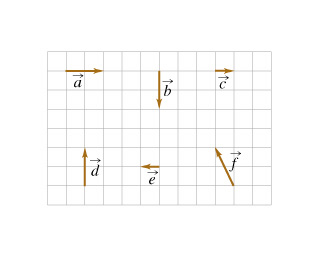# Problem: Six vectors (a through f ) have the magnitudes and directions indicated in the figure. (Figure 1)Rank the vector combinations on the basis of their angle, measured counterclockwise from the positive x-axis. Vectors parallel to the positive x-axis have an angle of 0°. All angle measures fall between 0° and 360°.Rank from largest to smallest. To rank items as equivalent, overlap them.a+df+ca+ba+ea+cd

###### FREE Expert Solution

Vector direction:

$\overline{){\mathbf{tan}}{\mathbf{\theta }}{\mathbf{=}}\frac{\mathbf{y}}{\mathbf{x}}}$

a + d = 2i + 2j

θa+d = tan-1 (y/x) = tan-1 (2/2) = 45°

f + c = -1i + 2j + 1i = 2j

The vector is pointing in the positive y-axis. Posive y-axis is directed 90° from the positive x-axis counter clockwise.

θf+c = 90°

a + b = 2i - 2j

97% (354 ratings)###### Problem Details

Six vectors (a through f ) have the magnitudes and directions indicated in the figure. (Figure 1)Rank the vector combinations on the basis of their angle, measured counterclockwise from the positive x-axis. Vectors parallel to the positive x-axis have an angle of 0°. All angle measures fall between 0° and 360°.

Rank from largest to smallest. To rank items as equivalent, overlap them.

a+d

f+c

a+b

a+e

a+c

d